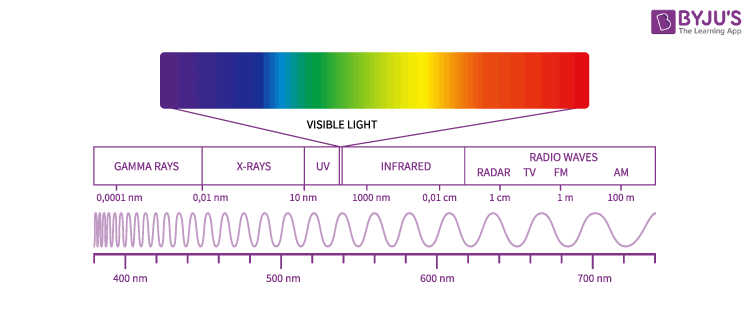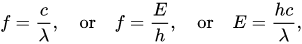# Electromagnetic Spectrum

Electromagnetic spectrum in simple terms is defined as the range of all types of electromagnetic radiation. We shall learn about the concept in detail and understand all its underlying aspects in this lesson.

## What are Electromagnetic Spectrum?

The electromagnetic spectrum is a range of frequencies, wavelengths and photon energies covering frequencies from below 1 hertz to above 1025 Hz corresponding to wavelengths which are a few kilometres to a fraction of the size of an atomic nucleus in the spectrum of electromagnetic waves. Generally, in a vacuum electromagnetic waves tend to travel at speeds which is similar to that of light. However, they do so at a wide range of wavelengths, frequencies, and photon energies.

The electromagnetic spectrum consists of a span of all electromagnetic radiation which further contains many subranges which are commonly referred to as portions. These can be further classified as infra-red radiation, visible light or ultraviolet radiation.

## Electromagnetic Waves in Electromagnetic Spectrum

The entire range (electromagnetic spectrum) is given by radio waves, microwaves, infrared radiation, visible light, ultra-violet radiation, X-rays, gamma rays and cosmic rays in the increasing order of frequency and decreasing order of wavelength. The type of radiation and their frequency and wavelength ranges are as follows:

 Type of Radiation Frequency Range (Hz) Wavelength Range gamma-rays 1020 – 1024 < 10-12 m x-rays 1017 – 1020 1 nm – 1 pm ultraviolet 1015 – 1017 400 nm – 1 nm visible 4 x 1014 – 7.5 x 1014 750 nm – 400 nm near-infrared 1 x 1014 – 4 x1014 2.5 μm – 750 nm infrared 1013 – 1014 25 μm – 2.5 μm microwaves 3 x 1011 – 1013 1 mm – 25 μm radio waves < 3 x 1011 > 1 mm

The electromagnetic spectrum can be depicted as follows :We see the uses of the electromagnetic waves in our daily life as :

Microwave: This type of radiation is found in microwaves and helps in cooking at home/office. It is also used by astronomers to determine and understand the structure of nearby galaxies and stars.

Infrared: It is used widely in night vision goggles. These devices can read and capture the infrared light emitted by our skin and objects with heat. In space, infrared light helps to map the interstellar dust.

X-ray: X-rays can be used in many instances. For example, a doctor can use an x-ray machine to take an image of our bone or teeth. Airport security personnel use it to see through and check bags. X-rays are also given out by hot gases in the universe.

Gamma-ray: It has a wide application in the medical field. Gamma-ray imaging is used to see inside our bodies. Interestingly, the universe is the biggest gamma-ray generator of all.

Ultraviolet: Sun is the main source of ultraviolet radiation. It causes skin tanning and burns. Hot materials that are in space also emit UV radiations.

Visible: Visible light can be detected by our eyes. Light bulbs, stars, etc. emit visible light.

Spectroscopy:

Spectroscopy is used to study the way different electromagnetic waves interact with matter.

We can learn about a substance by analyzing the EM spectrum given by it. When light scatters or passes through matter, it tends to interact with the molecules and atoms. Since atoms and molecules have resonance frequencies they directly interact with those light waves having the exact frequencies. When collisions occur in an excited state, the atoms and molecules emit light with a certain set of characteristic frequencies. This further results in a line spectrum. Here, only light with detached wavelengths is produced. The spectrum is also not continuous but it consists of a set of emission lines.

In cases where the light with continuous wavelengths passes through a low-density material, the atoms and molecules of the material will absorb light waves with the same set of characteristic frequencies. This results in the production of the absorption spectrum which is a nearly continuous spectrum with missing lines.

## Significance of Electromagnetic Spectrum

The electromagnetic waves in these different bands have different characteristics depending upon how they are produced, how they interact with matter and their practical applications. Maxwell’s equations predicted the existence of an infinite number of frequencies of electromagnetic waves, all travelling with the speed of light. This is the first indication of the existence of the entire electromagnetic spectrum.

Nonetheless, the main significance of the electromagnetic spectrum is that it can be used to classify electromagnetic waves and arrange them according to their different frequencies or wavelengths.

### Practical Applications of Electromagnetic Waves

● The radio waves and microwaves discovered by Hertz paved the way for wireless television and radio and mobile communication.

● The visible light portion of the electromagnetic spectrum is the reason for all visual aids in daily life. This is the portion of the electromagnetic spectrum that helps us to see all the objects, including the colours.

● The X-rays discovered by Roentgen proved to be useful in medicine for detecting many ailments or deformities in bones.

● The high ultraviolet radiation has energies to ionize the atoms causing chemical reactions.

● The gamma rays discovered by Paul Villard are useful for ionization purposes, and for nuclear medicine.

The frequency(f), speed(c), energy(E), wavelength(λ) of electromagnetic waves are related as:where:

c = 299792458 m/s is the speed of light in a vacuum

h = 6.62607015×10−34 J·s = 4.13566733(10)×10−15 eV·s is Planck’s constant.

## Solved Questions

Question 1: What are the frequency and wavelength of an EM wave of energy 6.626 x 10-19 J?

Answer: Frequency(f) = E/h = 1015 Hz. Wavelength(λ) = c/f = 3 x 108 / 1015 = 3 x 107 metres.

Question 2: What are the uses of X-rays?

Answer: X-rays can be used to detect medical ailments, bone deformities in medical diagnosis. They are useful for ionization purposes also.

Question 3: Can we use X-rays and gamma rays for broadcasting radio/TV/mobile signals?

Answer: No, the X-rays and gamma rays are short range. Moreover, they are harmful and have penetrating power on the matter with which they interact and can damage the tissues of living bodies.

Question 4: Which among the following is not a property of electromagnetic waves?

1) Momentum

2) Energy

3) Pressure

4) Heat energy

1) EM waves can impart momentum (and angular momentum) to the material with which it interacts.

2) Electromagnetic waves carry energy. EM waves are the only waves able to carry energy across a vacuum.

3) EM waves also exert pressure, which is shown by a radiometer. One side of the panels is black, the other side is white and the panels spin due to the pressure differences under the light.

4) EM waves do not carry heat energy but any EM radiation can heat an object when it is absorbed.

Question 5: A ray from the sun is passing through your kitchen window hits a prism that casts a rainbow on the windowsill. Supposedly, there is a hand-held radiometer on the table. Now you place the instrument on a specific colour of the rainbow with your eyes closed. When you open your eyes you see that the radiometer measured the energy from that colour at 4.0 x 10-19 joules. If we take Planck’s constant of 6.6256 x 10-34 joules/sec, what possible colour did the instrument measure? How can we determine this?

We should use the equation involving energy change, Planck’s constant, and frequency. Next, we need to figure out what we are solving for. In this problem, they ask for the possible colour that you measured. If we relate the energy with Planck’s constant, we can solve for frequency. We are given the energy, 4.0 x 10-19 joules, as well as Planck’s constant, 6.6256 x 10-34 joules/sec. Also, we are given the frequencies emitted by the visible spectrum, from red to violet. This problem is easy to solve now. If we solve for the frequency, we can then relate it to the energy emitted, measured in either sec-1.

Let’s solve:

– What is the best equation to use? E = h* ƒ

– Solve for the intended variable. E/ h = ƒ

– Energy measured = 4.0 x 10-19 joules

Planck’s constant = 6.6256 x 10-34 joules/sec

Visible spectrum frequency:

(4.0 x 10-19 joules) / 6.6256 x 10-34 joules/sec = ƒ

– Joules cancel out with joules, and one is left with sec-1, a frequency.

Answer = 6.03 x 1014 sec-1

This falls within the given visible spectrum frequencies. Being a high frequency on the visible spectrum, close to the frequencies emitted by blue colour, one could assume that you measured a colour close to blue; though cyan and green cannot be out of the question as the given frequencies are a bit vague. It is closest to the green colour. Hence, you measured the green colour.

## Rutherford’s Model and the Electromagnetic Spectrum## Frequently Asked Questions on Electromagnetic Spectrum

### Which electromagnetic spectrum has the highest wavelength?

Electromagnetic spectrum is a collection of a range of waves in a sequential order. The radio waves have the highest frequency and the gamma rays have the shortest wavelength.

### Which part of the electromagnetic spectrum carries the least energy?

Radio waves have the lowest frequency and hence have the least energy.
E = hf
E = energy
f = frequency
h = Planck’s constant

### Where in the electromagnetic spectrum is visible light?

Visible light falls in the range of the electromagnetic spectrum between infrared rays and ultraviolet rays. It has frequencies of about 4 × 1014 to 8 × 1014 hertz (Hz) and a wavelength of about 740 nanometers ( 2.9 × 10−5 inches) to 380 nanometers (1.5 × 10−5 inches).

### Which part of the electromagnetic spectrum has the highest frequency?

Gamma rays have the highest frequency and the least wavelength.

Test your Knowledge on electromagnetic spectrum and electromagnetic waves